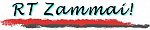Sitemap | Coverstory | Photos | Railways z. | Bridges z. | Seeds
\$B8=:_\$N%Z!<%8(B :
 \$B%H%C%W(B >> \$B\$6\$s\$^\$\$\$4>R2p(B >> \$B\$*\$^\$1(B2
You are now in :
 Top >> "Zammai" Introduction >> "Bonus" 2

\$B:G=*99?7(B: 2005. 01. 02.
Last updated: 02 January 2005

# \$B\$*\$^\$1(B: \$B\$h\$&\$3\$=!"(BRT\$B\$6\$s\$^\$\$\$X(BBonus article: Welcome to the "RT Zammai"

 This is an old greeting article for greetings, which was written upon startup of RT Zammai web site. It seems that, at the beginning, I was not sure of what to write as contents. I even seemed to have thought that this cannot be continued. I have read a newspaper article pointing out that many people who try to open their own web sites tend to face the same problem. But, things have carried on for two years! Fear is often greater than the danger... No English translation of the essay is available.
\$B!V(BRT\$B\$6\$s\$^\$\$!W\$rN)\$A>e\$2\$?\$H\$-\$K=q\$\$\$?0';"J8\$G\$"\$k!#(B

\$B\$I\$&\$d\$i!"(B \$B2?\$r=q\$\$\$?\$i\$\$\$\$\$+G:\$s\$G\$\$\$?\$h\$&\$G\$"\$k!#(B \$BB3\$1\$k\$3\$H\$,\$G\$-\$J\$\$\$s\$8\$c\$J\$\$\$+!"(B \$B\$H\$b=q\$\$\$F\$"\$k\$+\$i!"(B \$BAjEv<+?.\$,\$J\$+\$C\$?\$h\$&\$G\$"\$k!#(B

\$B \$B\$7\$+\$7!"(B \$B\$o\$,(BRT\$B\$6\$s\$^\$\$\$b\$^\$b\$J\$/(B2\$BG/!#(B \$B0F\$:\$k\$h\$j@8\$`\$,\$d\$9\$7!"(B \$B\$H\$\$\$C\$?\$H\$3\$m\$@\$m\$&\$+!#(B

\$BK\%Z!<%8\$K%"%/%;%9\$7\$F\$/\$@\$5\$C\$?FIe\$2\$^\$9!#(B

\$B\$3\$N%Z!<%8\$O!"(B \$B9bLZ!!N<(B\$B\$N8D?ME*\$J%&%'%V!&%Z!<%8\$G\$9!#(B

#### \$B%?%\$%H%k!V(BRT\$B\$6\$s\$^\$\$!W\$NM3Mh(B \$B!A%a%\$%s%3%s%;%W%H\$O!V=P\$7B3\$1\$k!W!A(B

\$BK\%Z!<%8\$N%?%\$%H%k!V(BRT\$B\$6\$s\$^\$\$!W\$K\$O!"(B \$B=P\$7!VB3\$1!W\$k\$3\$H\$r\$h\$j6/\$/G0F,\$K\$*\$\$\$F!"(B \$B9bLZ\$,9%\$-J|Bj\$G\$-\$k6u4V!"(B \$B\$H\$\$\$&5\$;}\$A\$r9~\$a\$^\$7\$?!#(B \$B\$3\$l\$O!"(B \$B99?7\$5\$l\$J\$\$%&%'%V%Z!<%8\$N>pJs\$O!"(B \$B99?7\$5\$l\$J\$\$\$H\$\$\$&0lE@\$G\$=\$NIJ \$B\$A\$J\$_\$K!"!V(BRT\$B!W\$O!V9bLZ!!N\$O%U%!!<%9%H%M!<%`\$H%i%9%H%M!<%`\$rF|K\8l\$N=g=x\$GI=5-\$7\$F\$"\$j\$^\$9\$,!"(B \$B%\$%K%7%"%k\$O%U%!!<%9%H%M!<%`\$r@h\$K\$7\$?(B Ryo Takagi \$B\$NN,\$G\$"\$k(B RT \$B\$r;H\$\$\$J\$l\$F\$\$\$k\$N\$G!"\$3\$&\$7\$F\$"\$k!K!#(B \$B!V\$6\$s\$^\$\$(B!\$B!W\$O4A;z\$G\$O!V;0Kf!W\$H=q\$/8@MU\$G\$"\$j!"(B \$B7h\$7\$F(B \$B\$I\$3\$>\$N%3%s%T%e!<%?;(;o\$NM-L>\$JO":\5-;v(B\$B\$H\$O4X78\$4\$6\$\$\$^\$;\$s!#(B(\$B>P(B)

#### \$BR2p(B

\$B\$7\$+\$7!"(B \$B9%\$->!pJs\$H\$9\$k\$Y\$/EXNO\$9\$k\$D\$b\$j\$G\$9!#(B \$B%U%m%s%H%Z!<%8\$K\$O!"(B \$B0l1~=5BX\$o\$j\$/\$i\$\$\$NM=Dj\$GC;\$\$J8>O\$r=P\$7B3\$1\$h\$&\$H9M\$(\$F\$\$\$^\$9!#(B \$B!V(B\$B:#=5\$N(B\$B4,F,8@!W\$H\$\$\$&\$o\$1\$G\$9!#(B \$B!V(BRT\$B\$6\$s\$^\$\$(B!\$B!W\$N5pBg%m%4\$N\$o\$-\$K\$b!"(B \$B\$3\$l\$b=5BX\$o\$jDxEY\$NM=Dj\$G \$B%Z!<%8\$NCf?H\$O!"(B \$B<+8J>R2p%Z!<%8(B \$B\$K\$"\$kI.pJs\$+\$i\$"\$kDxEY?d;!\$5\$l\$k\$h\$&\$JFbMF\$K\$J\$k\$G\$7\$g\$&!#(B \$BH>J,;E;v!"H>J,O\$rFI\$`!"2;3Z\$rJ9\$/!"1G2h\$r8+\$k!"\$J\$I\$\$\$m\$\$\$m!K(B \$B\$+\$i9M\$(\$?\$3\$H\$r5-=R\$7\$?%3%s%F%s%D\$J\$I\$r=8\$a\$F\$\$\$3\$&\$H9M\$(\$F\$\$\$^\$9!#(B
##### \$B\$40U8+\$*J9\$+\$;2<\$5\$\$(B
\$B!V=P\$7B3\$1\$k!W\$,%a%\$%s%3%s%;%W%H\$N%Z!<%8\$G\$9\$+\$i!"(B \$B1J1s\$K!V9);vCf!W\$N%Z!<%8\$G\$b\$"\$j\$^\$9!#(B \$B7G<(HD\$NN`\$\$\$OEvLLMQ0U\$;\$:!"(B \$B9bIJpJs\$r<+J,\$N@UG\$\$K\$*\$\$\$F5-=R\$7!"=8\$a\$k\$3\$H\$rL\;X\$9\$D\$b\$j\$G\$9\$,!"(B \$BFIpEy!"(B \$B\$*5\$7Z\$KEE;R%a!<%k\$K\$F\$*4s\$;\$/\$@\$5\$\$\$^\$9\$h\$&\$*4j\$\$?=\$7>e\$2\$^\$9!#(B
\$B9bLZ(B \$BN<(B / TAKAGI, Ryo webmaster@takagi-ryo.ac